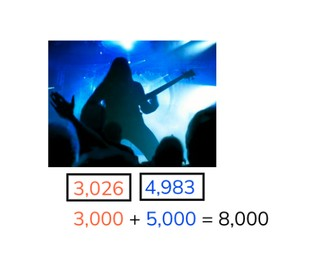Estimate the sum up to 10,000

# Estimate the sum up to 10,000

Estimate the sum to 10,0008,000 schools use Gynzy92,000 teachers use Gynzy1,600,000 students use Gynzy

## General

Students learn to estimate the sum of numbers up to 10,000 by rounding to the nearest hundred or thousand.

## Standards

CCSS.Math.Content.4.NBT.B.4

## Learning objective

Students will be able to estimate the sum up to 10,000.

## Introduction

Practice rounding numbers by asking students which thousands number the given numbers are closest to when using the number line. Then look at the different objects and ask students about what they cost together as well as what they exactly cost together. Discuss with students how you round the numbers.

## Instruction

Explain to students that with estimated sums you first round the numbers and then solve the problem. The problem of 3,998 + 1,984 for example can be solved by using the estimated problem of 4,000 + 2,000. With another example show students that you can round the numbers to a hundreds or thousands number. Discuss the statements about rounding to hundreds or thousands numbers. Remind students that the way you round makes a difference to your estimate. If you want to stay closer to the exact sum, you can round to a closer number (say a hundreds number), rather than rounding to the nearest thousand. Practice estimated sums by asking students to match the best estimated problem for the three given problems. Then practice estimating the sum and determining if the total is more or less than the exact sum. Ask students to explain their strategy. Finally practice estimation in context that students might recognize.

To check that students are able to estimate the sum to 10,000 ask them the following questions:
- Why is it useful to be able to estimate the sum?
- If you round the numbers as large as possible, are you closer to the exact sum or further away from it?

## Quiz

Students are given a set of ten questions in which they are asked to determine which estimated sum belongs to a given problem, to estimate the sum, to estimate what range a sum would fall into and to solve the estimated and exact sums.

## Closing

Discuss with students why it is important to be able to estimate. It is useful if you want to be able to figure out about how much you have. In addition you can use it when you solve problems exactly, because it can help you check your work as you solve, since you already know about how much the total should be. Next on the board you are given three numbers. Students must determine which numbers match if you round to the tens, hundreds, and thousands. You can drag these numbers to where they belong. Discuss how you round a number, and remind students which number they should look at when rounding to the tens, hundreds, and thousands. Finally estimate the sum of the given problems. Discuss the problems in groups and as a class.

## Teaching tips

Students who have difficulty with this learning goal can be supported by practicing rounding numbers. You can use a number line to help them visualize whether they need to round up or down with a given number. Once they are comfortable with rounding, give them numbers that are easier to round to build proficiency. You can also discuss strategic rounding with the students. With the number 2,896 is most precise when rounded to 2,900, but for a quick estimation it is easiest to round the number to 3,000.

### The online teaching platform for interactive whiteboards and displays in schools

• Save time building lessons

• Manage the classroom more efficiently

• Increase student engagement